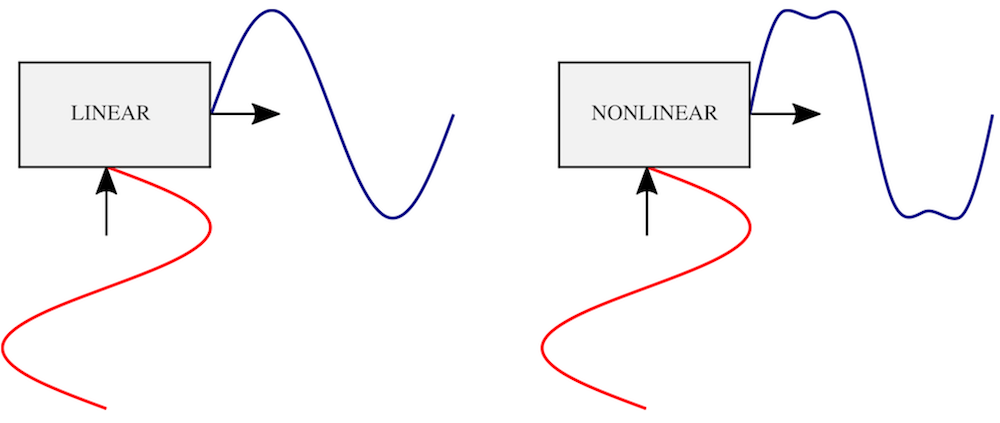# 扬声器驱动器的非线性失真分析

2018年 6月 18日

### 认识线性和非线性失真1. 线性失真
2. 非线性失真{in} \sin \left( 2\pi f t \right)

\mathrm{THD} = \frac{\sqrt{A_\text{out}^2 \left( 2f \right) + A_\text{out}^2 \left( 3f \right) + A_\text{out}^2 \left( 4f \right) + \dots}}{A_\text{out} \left( f \right)} \times 100\%.

\sin \left( 2\pi f_1 t \right) + B_\text
{in} \sin \left( 2\pi f_2 t \right)
（其中 f_2 > f_1）的双频正弦波应用于输入，系统非线性可导致较高频率分量被较低的频率分量调制。也就是说，f_2 \pm f_1f_2 \pm 2f_1 等频率将出现在输出信号的频谱中。频率 f_2 \pm (n-1) f（其中 n \geq 2）对应的互调的定量测量值是 nth 阶互调失真（intermodulation distortion，简称 IMD）系数，其定义为：

\mathrm{IMD}_n = \sqrt{ \frac{ B_\text{out}^2 \left( f_2 + ( n-1 ) f_1 \right) + B_\text{out}^2 \left( f_2-( n-1 ) f_1 \right)}{A_\text{out}^2 \left( f_1 \right) + B_\text{out}^2 \left( f_2 \right)}} \times 100\%.

### 扬声器驱动器的瞬态非线性分析

• 扬声器的非线性行为如何影响输出信号？
• 确保扬声器正常运行的输入信号的极限是什么？
• 应该如何补偿扬声器的不良失真？

V_\text{in}
\left( t \right) = V_0 \sin \left( 2 \pi f t \right)

V_\text
{in}
\left( t \right) = V_1 \sin \left( 2\pi f_1 t \right) + V_2 \sin \left( 2\pi f_2 t \right)

V_1V_2 的大小通常为 4 : 1，相当于 12 dB。

### 分析扬声器驱动器的建模技巧

• 由高磁导率金属制成的扬声器极片的磁场的非线性行为
• 电机活动部件的几何非线性
• 当音圈在气隙中上下移动时的拓扑变化

### 后续操作

#### 更多资源

• 查看相关教程中的扬声器建模示例：
• 延伸阅读：
• L.L. Beranek and T.J. Mellow, Acoustics: Sound Fields and Transducers, Academic Press, 2012.
• Brüel & Kjær, “Audio Distortion Measurements,” Application Note BO0385, 1993.
• W. Marshall Leach, Jr., Introduction to Electroacoustics and Audio Amplifier Design, Kendall Hunt, 2010.